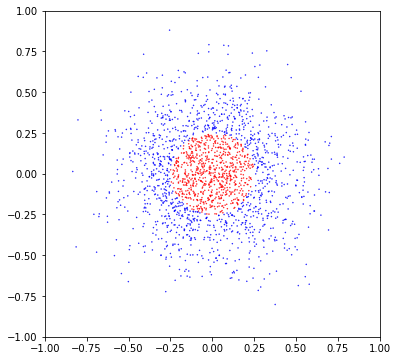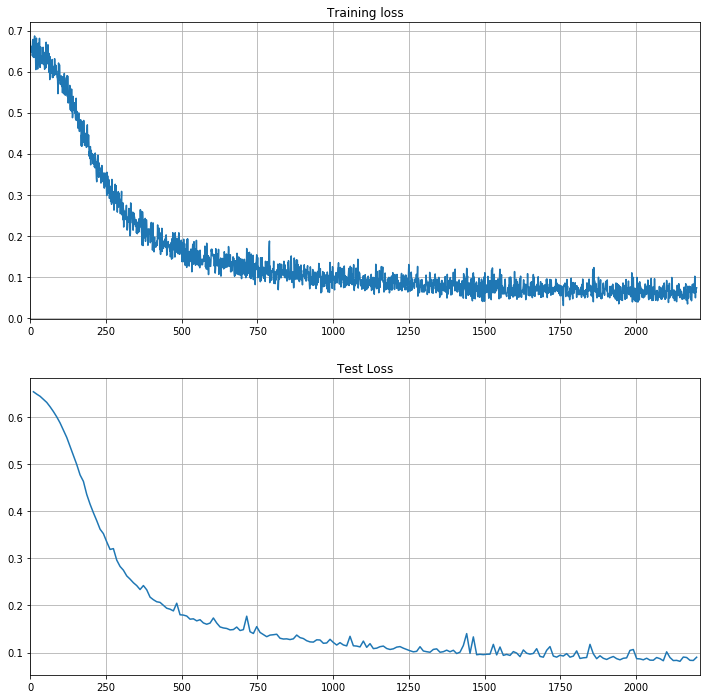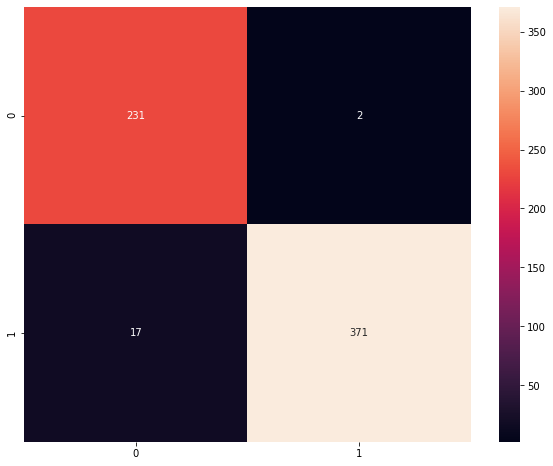# Hands-On: Simple Feed Forward Neural Network in BigDL¶

## Generating the Data¶

For this example we will generate a simple dataset to train our first BigDL full fledged neural network. Let's start by importing the necessary packages for number manipulation and visualization and setting up a seed for the RGN:

In :
import numpy as np
import numpy.random as rd
import pandas as pd
import matplotlib.pyplot as plt
import seaborn as sns

# Set up a seed for the RNG
seed = 2019
rd.seed(seed)


As we have already worked with the logistic regression, so this time we would like to see how the neural network performs in non-linearly separable data:

In :
# Define data parameters
num_features = 2
num_classes = 2
num_samples = 2000

mean = 0.0
var = 0.26
threshold = 0.25

x = rd.normal(mean, var, num_samples)
y = rd.normal(mean, var, num_samples)
labels = np.array([np.linalg.norm(s) > threshold for s in zip(x,y)])
colors = ['r', 'b']

plt.figure(figsize=(6,6))
plt.scatter(x,y, c=[colors[int(l)] for l in labels], s=0.2);
plt.xlim(-1,1); plt.ylim(-1,1);This data is obviously non-separable by a logistic regression (at least without transforming the feature vectors x and y).

In :
from bigdl.util.common import *
from pyspark import SparkContext
# Get the spark context
sc = SparkContext.getOrCreate(conf=create_spark_conf().setMaster("local").set("spark.driver.memory","10g"))
samples = [Sample.from_ndarray(np.array([xx,yy]), np.array(l)+1) for xx,yy,l in zip(x,y,labels)]
samples_rdd = sc.parallelize(samples)
train_test_rate = 0.7

train_rdd, test_rdd = samples_rdd.randomSplit([train_test_rate, 1-train_test_rate], seed)
print("Train observations: {}".format(train_rdd.count()))
print("Test observations: {}".format(test_rdd.count()))

Train observations: 1379
Test observations: 621


## Initializing the BigDL engine¶

We need to initialize the BigDL engine before doing any BigDL work as usual:

In :
init_engine()


## Building the Feed Forward Neural Network¶

We will now define a very basic feed forward neural network which we will use to classify the dataset. It will consists of two layers, a first Linear layer with ReLU activation and a second Linear layer with LogSoftMax activation. For illustration purposes we will show how to define them using both the Sequential and Functional APIs:

### Sequential API¶

In :
from bigdl.nn.layer import *

num_hidden = 10

ff_seq = Sequential()
print(ff_seq)

creating: createSequential
creating: createLinear
creating: createReLU
creating: createLinear
creating: createLogSoftMax
Sequential{
[input -> (1) -> (2) -> (3) -> (4) -> output]
(1): Linear[88e612da](2 -> 10)
(2): ReLU[51ed065](0.0, 0.0)
(3): Linear[ba5b58bd](10 -> 2)
(4): LogSoftMax[aefa83ea]
}


### Functional API¶

In :
lin1 = Linear(num_features, num_hidden)()
relu = ReLU()(lin1)
lin2 = Linear(num_hidden, num_classes)(relu)
sigmoid = Tanh()(lin2)
ff_fun = Model(lin1, sigmoid)
for l in ff_fun.layers:
print(l)

creating: createLinear
creating: createReLU
creating: createLinear
creating: createTanh
creating: createModel
Linear[1418ed31](2 -> 10)
ReLU[be4b0bda](0.0, 0.0)
Linear[b6df3fa](10 -> 2)
Tanh[800f6d19]


## Training the model¶

As we saw in the logistic regression notebook, for classification problems the recommended optimization criterion is ClassNLLCriterion. We create the Optimizer as usual:

In :
from bigdl.optim.optimizer import *
from bigdl.nn.criterion import *
from bigdl.optim.optimizer import *

batch_size = 128
epochs = 200
learning_rate = 0.4

optimizer = Optimizer(
model=ff_seq,
training_rdd=train_rdd,
criterion=ClassNLLCriterion(),
end_trigger=MaxEpoch(epochs),
optim_method=SGD(learningrate=learning_rate),
batch_size=batch_size)

creating: createClassNLLCriterion
creating: createMaxEpoch
creating: createDefault
creating: createSGD
creating: createDistriOptimizer


To be able to trace the training progress we add validation and enable logging:

In :
# Define a validator for the Optimizer
optimizer.set_validation(
batch_size=batch_size,
val_rdd=test_rdd,
trigger=EveryEpoch(),
val_method=[Loss()]
)

# Define the Logs and pass them to the Optimizer
import datetime as dt
log_dir = '/tmp/bigdl_summaries'
app_name = 'nn-ff-' + dt.datetime.now().strftime("%Y%m%d-%H%M%S")

# Create the train and validation summaries
train_summary = TrainSummary(log_dir=log_dir, app_name=app_name)
val_summary = ValidationSummary(log_dir=log_dir, app_name=app_name)

# Pass them to the optimizer
optimizer.set_train_summary(train_summary)
optimizer.set_val_summary(val_summary)
print("Logs saved to: {}/{}".format(log_dir, app_name))

creating: createEveryEpoch
creating: createClassNLLCriterion
creating: createLoss
creating: createTrainSummary
creating: createValidationSummary
Logs saved to: /tmp/bigdl_summaries/nn-ff-20200204-173636


And finally train the model:

In :
optimizer.optimize();


## Validating the model¶

Let's plot the loss information recorded in the training and validation logs:

In :
import matplotlib.pyplot as plt

plt.figure(figsize = (12,12))
plt.subplot(2,1,1)
plt.plot(loss_train[:,0],loss_train[:,1],label='Training loss')
plt.xlim(0,loss_train.shape+10)
plt.grid(True)
plt.title("Training loss")

plt.subplot(2,1,2)
plt.plot(loss_test[:,0],loss_test[:,1],label='Test loss')
plt.xlim(0,loss_train.shape+10)
plt.title("Test Loss")
plt.grid(True)And plot the confusion matrix:

In :
from sklearn.metrics import confusion_matrix
from sklearn.metrics import accuracy_score
import pandas as pd

# Remember that labels are 1-indexed in BigDL:
y_pred = np.array(ff_seq.predict_class(test_rdd).collect())-1
y_label = np.array([s.label.to_ndarray() - 1 for s in test_rdd.collect()])

acc = accuracy_score(y_label, y_pred)
print("The prediction accuracy is %.2f%%"%(acc*100))

cm = confusion_matrix(y_label, y_pred)
df_cm = pd.DataFrame(cm)
plt.figure(figsize = (10,8))
sns.heatmap(df_cm, annot=True,fmt='d');

The prediction accuracy is 96.94%